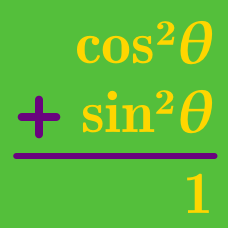Geometry

# Pythagorean Identities: Level 4 Challenges

$\begin{cases} \cos \alpha = \tan \beta \\ \cos \beta = \tan \gamma \\ \cos \gamma = \tan \alpha \end{cases}$

The acute angles $\alpha, \beta$ and $\gamma$ satisfy the system of equations above. Find $\sin \gamma$.

Give your answer to 2 decimal places.

Find the value of $E=\tan^2{\dfrac{\pi}{40}}+\tan^2{\dfrac{3\pi}{40}}+\tan^2{\dfrac{5\pi}{40}}+\cdots+\tan^2{\dfrac{19\pi}{40}}.$

$\begin{cases} \dfrac{\sin\theta}{x} =\dfrac{\cos\theta}{y} \\ \dfrac{\cos^{4}\theta}{x^{4}}+\dfrac{\sin^{4}\theta}{y^{4}}=\dfrac{97\sin 2\theta}{x^{3}y+y^{3}x} \end{cases}$

Let $x$ and $y$ be positive real numbers and $\theta$ is an angle such that it is not a multiple of $\frac{\pi}{2}$. If $x,y$ and $\theta$ satisfy the system of equations above, find $\dfrac{x}{y}+\dfrac{y}{x}$.

###### Source: 2009 Harvard-MIT Mathematics Tournament

For all real values of $\theta$ for which $\lvert \sin \theta \rvert \neq 1,$ evaluate:

$\large \sum_{n = 1}^{\infty} \sin^{2n}\theta .$

For all real values of $\theta$ for which $\lvert \sin \theta \rvert \neq 1,$ evaluate:

$\large \sum_{n = 1}^{\infty} \sin^{2n}\theta .$

×

Problem Loading...

Note Loading...

Set Loading...